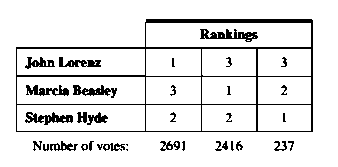# Election Three candidates are running for mayor. A vote was taken in which the candidates were ranked in order of preference. The results are shown in the preference schedule below. a. Use the Borda count method to determine the winner of the election. b. Verify that the majority criterion has been violated. c. Identify the candidate who wins all head-to-head comparisons. d. Explain why the Condorcet criterion has been violated. e. If Marcia Beasley drops out of there for mayor (and voter preferences remain the same), determine the winner of the election again, using the Borda count method. f. Explain why the independence of irrelevant alternatives criterion has been violated.### Mathematical Excursions (MindTap C...

4th Edition
Richard N. Aufmann + 3 others
Publisher: Cengage Learning
ISBN: 9781305965584

#### Solutions

Chapter
Section### Mathematical Excursions (MindTap C...

4th Edition
Richard N. Aufmann + 3 others
Publisher: Cengage Learning
ISBN: 9781305965584
Chapter 4.2, Problem 37ES
Textbook Problem
34 views

## Election Three candidates are running for mayor. A vote was taken in which the candidates were ranked in order of preference. The results are shown in the preference schedule below.a. Use the Borda count method to determine the winner of the election.b. Verify that the majority criterion has been violated.c. Identify the candidate who wins all head-to-head comparisons.d. Explain why the Condorcet criterion has been violated.e. If Marcia Beasley drops out of there for mayor (and voter preferences remain the same), determine the winner of the election again, using the Borda count method.f. Explain why the independence of irrelevant alternatives criterion has been violated.

To determine

(a)

Find the winner of the election by the help of Borda count method.

### Explanation of Solution

Given:

The given data is

Calculation:

Given:

Use Borda count method.

For each first place vote receive three points.

For each second place vote receive two points.

For each third place vote receive one point.

Vote received by Jhon Lorenz is,

First place vote =2691×3=8073

Second place vote =0×2=0

Third place vote =2653×1=2653

To determine

(b)

Check whether the majority criteria is violated or not.

To determine

(c)

To determine

(d)

Explain why the Condorcet criterion has been violated.

To determine

(e)

Find the winner of the election by the help of Borda count method.

To determine

(f)

Describe the independence of irrelevant alternatives criterion has been violated.

### Still sussing out bartleby?

Check out a sample textbook solution.

See a sample solution

#### The Solution to Your Study Problems

Bartleby provides explanations to thousands of textbook problems written by our experts, many with advanced degrees!

Get Started

Find more solutions based on key concepts
In Exercises 15-28, construct a truth table for the compound proposition. (pq)r

Finite Mathematics for the Managerial, Life, and Social Sciences

Divide: (800)(+25)

Elementary Technical Mathematics

In Exercises 29-34, find an equation of the circle that satisfies the given conditions. 34. Center (a, a) and r...

Applied Calculus for the Managerial, Life, and Social Sciences: A Brief Approach

Simplify 4(3)25(2)2.

Intermediate Algebra

Convert the expressions in Exercises 6584 to power form. 3x25472x3

Finite Mathematics and Applied Calculus (MindTap Course List)

Reminder Round all answers to two decimal places unless otherwise indicated. A Skydiver When a skydiver jumps f...

Functions and Change: A Modeling Approach to College Algebra (MindTap Course List)

ln |2x + 1| + C 2 ln |2x + 1| + C

Study Guide for Stewart's Single Variable Calculus: Early Transcendentals, 8th